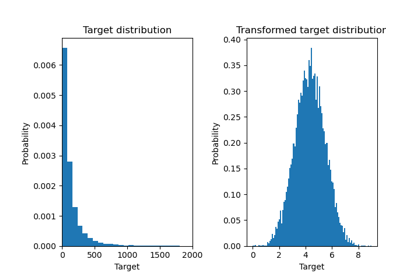# sklearn.preprocessing.quantile_transform¶

sklearn.preprocessing.quantile_transform(X, *, axis=0, n_quantiles=1000, output_distribution='uniform', ignore_implicit_zeros=False, subsample=100000, random_state=None, copy=True)[source]

Transform features using quantiles information.

This method transforms the features to follow a uniform or a normal distribution. Therefore, for a given feature, this transformation tends to spread out the most frequent values. It also reduces the impact of (marginal) outliers: this is therefore a robust preprocessing scheme.

The transformation is applied on each feature independently. First an estimate of the cumulative distribution function of a feature is used to map the original values to a uniform distribution. The obtained values are then mapped to the desired output distribution using the associated quantile function. Features values of new/unseen data that fall below or above the fitted range will be mapped to the bounds of the output distribution. Note that this transform is non-linear. It may distort linear correlations between variables measured at the same scale but renders variables measured at different scales more directly comparable.

Read more in the User Guide.

Parameters:
X{array-like, sparse matrix} of shape (n_samples, n_features)

The data to transform.

axisint, default=0

Axis used to compute the means and standard deviations along. If 0, transform each feature, otherwise (if 1) transform each sample.

n_quantilesint, default=1000 or n_samples

Number of quantiles to be computed. It corresponds to the number of landmarks used to discretize the cumulative distribution function. If n_quantiles is larger than the number of samples, n_quantiles is set to the number of samples as a larger number of quantiles does not give a better approximation of the cumulative distribution function estimator.

output_distribution{‘uniform’, ‘normal’}, default=’uniform’

Marginal distribution for the transformed data. The choices are ‘uniform’ (default) or ‘normal’.

ignore_implicit_zerosbool, default=False

Only applies to sparse matrices. If True, the sparse entries of the matrix are discarded to compute the quantile statistics. If False, these entries are treated as zeros.

subsampleint, default=1e5

Maximum number of samples used to estimate the quantiles for computational efficiency. Note that the subsampling procedure may differ for value-identical sparse and dense matrices.

random_stateint, RandomState instance or None, default=None

Determines random number generation for subsampling and smoothing noise. Please see subsample for more details. Pass an int for reproducible results across multiple function calls. See Glossary.

copybool, default=True

Set to False to perform inplace transformation and avoid a copy (if the input is already a numpy array). If True, a copy of X is transformed, leaving the original X unchanged

..versionchanged:: 0.23

The default value of copy changed from False to True in 0.23.

Returns:
Xt{ndarray, sparse matrix} of shape (n_samples, n_features)

The transformed data.

QuantileTransformer

Performs quantile-based scaling using the Transformer API (e.g. as part of a preprocessing Pipeline).

power_transform

Maps data to a normal distribution using a power transformation.

scale

Performs standardization that is faster, but less robust to outliers.

robust_scale

Performs robust standardization that removes the influence of outliers but does not put outliers and inliers on the same scale.

Notes

NaNs are treated as missing values: disregarded in fit, and maintained in transform.

Warning

Risk of data leak

Do not use quantile_transform unless you know what you are doing. A common mistake is to apply it to the entire data before splitting into training and test sets. This will bias the model evaluation because information would have leaked from the test set to the training set. In general, we recommend using QuantileTransformer within a Pipeline in order to prevent most risks of data leaking:pipe = make_pipeline(QuantileTransformer(), LogisticRegression()).

For a comparison of the different scalers, transformers, and normalizers, see examples/preprocessing/plot_all_scaling.py.

Examples

>>> import numpy as np
>>> from sklearn.preprocessing import quantile_transform
>>> rng = np.random.RandomState(0)
>>> X = np.sort(rng.normal(loc=0.5, scale=0.25, size=(25, 1)), axis=0)
>>> quantile_transform(X, n_quantiles=10, random_state=0, copy=True)
array([...])


## Examples using sklearn.preprocessing.quantile_transform¶Effect of transforming the targets in regression model

Effect of transforming the targets in regression model